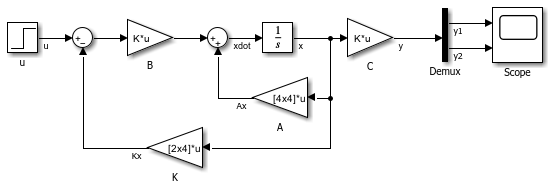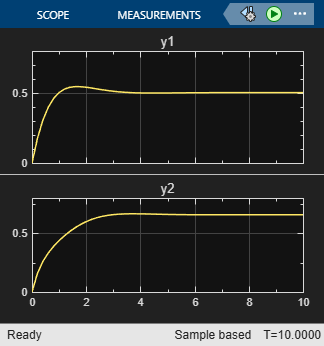# Extract Output Elements of Feedback System

This example demonstrates how to extract the output elements of a state-space system that uses vector signals.

Open the example model.These equations define the state-space system:To ensure that the system is stable, the state feedback vectoris chosen such that the eigenvalues ofequal,,, and.

The Demux block extracts the two elements from the output vector. Then, the Scope block separately plots each element it receives.